# Experimental and Three-Dimensional Investigation on Thermal Performance of a Vortex Tube

Experimental and Three-Dimensional Investigation on Thermal Performance of a Vortex Tube
Department of Mechanical Engineering, Urmia University of Technology, Urmia, Iran

Corresponding Author Email:
n.geramian@mee.uut.ac.ir
Page:
21-27
|
DOI:
https://doi.org/10.18280/psees.030104
5 October 2019
|
Accepted:
25 December 2019
|
Published:
30 December 2019
| Citation

OPEN ACCESS

Abstract:

Energy separation procedure of vortex tube can be improved by using truncated cone throttle valve. Experiments are performed to study the effect of the geometrical parameters on vortex tube refrigeration capacity by using air as the working fluid. In this experimental investigation, the parameters are focused on the cone length of throttle valve, inlet pressure and number of nozzle intakes. The effect of the cone length of throttle valve is also investigated in the range from 0 to 10 mm. The results present that there is an optimum cone length for obtaining the highest efficiency and φ=6mm is the optimal candidate under our experiments. A computational fluid dynamics model was developed to predict the performances of the vortex tube system. The numerical investigation was carried out by full 3D steady state CFD-simulation using FLUENT 6.3.26. This model utilizes the k-ε turbulence model to solve the flow equations.

Keywords:

experimental study, numerical simulation, vortex tube, cone length, truncated cone

1. Introduction

The vortex tube is a device that has a simple geometry, without any moving complicated parts or external energy supply that separates a pressurized gas into hot and cold streams. A schematic drawing of a typical vortex tube and its proceeds is shown in Figure 1. A vortex tube includes different parts such as: one or more inlet nozzles, a vortex-chamber, a cold end orifice, a control valve that is located at hot end and a working tube. When pressured gas is injected into the vortex-chamber tangentially via the nozzles, a strong rotational flow field is established.

## 1.jpg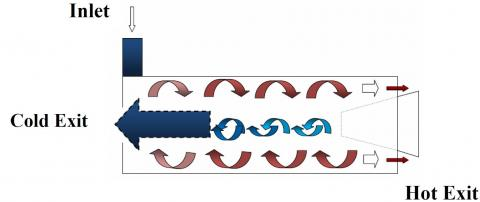Figure 1. A schematic form of simple vortex tube

When the gas swirls to the center of the vortex-chamber it is expanded and cooled. After occurrence of the energy separation procedure in the vortex tube the pressured inlet gas stream was separated into two different gas streams including cold and hot exit gases. The “cold exit or cold orifice” is located at near the inlet nozzle and at the other side of the working tube there is a changeable stream restriction part namely the conical control valve which determines the mass flow rate of hot exit. As seen in Fig. 1, a percent of the compressed gas escapes through the conical valve at the end of the tube as hot stream and the remaining gas returns in an inner swirl flow and leaves through the cold exit orifice. Opening the hot control valve reduces the cold airflow and closing the hot valve increases the cold mass flow rate.

Cold mass fraction or α can been defined as below:

$\alpha=\frac{\dot{m}_{c}}{\dot{m}_{i}}$   (1)

In this equation $\dot{m}_{c}$ is the mass flow rate at the cold exit and $\dot{m}_{i}$ is the inlet flow rate. Vortex tube performance was first discovered by Ranque  when he was investigating processes in a dust separation cyclone. The German physicist Hilsch  improved the design of this mechanism. Some of investigations on various aspects of vortex tubes are briefly mentioned below: Aydın and Baki  carried out an experimental study on the design parameters of a counter flow vortex tube. In this experimental investigation, the designed parameters and performances of counter flow vortex tubes are studied. Under different inlet pressures, the thermal performance as a function of the geometrical parameters is investigated including the vortex tube length, the inlet nozzle diameter, and the angle of the hot control valve. Also, three different working gases are employed; air, oxygen, and nitrogen. Kurosaka  stated that the temperature separation is a result of acoustic streaming effect. Stephan et al.  proposed the formation of Gortler vortices on the inside wall of the vortex tube that drive the fluid motion. Dincer et al.  experimentally investigated the distinction between the hot and cold streams of vortex tubes with different length to diameter ratios (L/D). Ahlborn and Gordon  explained an embedded secondary circulation. Akhesmeh et al.  made a 3D CFD model to study the variation of velocity, pressure, and temperature inside a vortex tube. Their results obtained upon the numerical approach comprehensively emphasized on the mechanism of hot peripheral flow and a reversing inner cold core flow formation. Aljuwayhel et al.  utilized a fluid dynamics model of a vortex tube to realize the procedure of energy separation phenomena. Skye et al.  employed a model similar to that of Aljuwayhel et al. . Rafiee et al.  numerically investigated the effect of the working tube radius and importance of the stagnation point occurrence inside the vortex tube. Chang  performed an experimental investigation of vortex tube refrigerator with a divergent hot tube. In their work, the parameters are focused on some geometrical parameters such as divergence angle of hot tube, length of divergent hot tube, and number of nozzle intakes. Experimental results show that there is an optimal angle to achieve the highest refrigeration capacity. Aydin and Baki  carried out an experimental study on the design parameters of a counter flow vortex tube. In their investigation, the design parameters and performances of counter flow vortex tubes are studied. Under different inlet pressures, the thermal performance as a function of the geometrical parameters is investigated including the vortex tube length, the inlet nozzle diameter, and the angle of the hot control valve. Moreover, three different working system gases are employed; air, oxygen, and nitrogen. Im and Yu  investigated the effect of the nozzle area ratio on the performance of vortex tube with different inlet pressures. Polat and Kirmaci  presented a work focused on determination of gas types in counter flow type vortex tubes. In their study, four different gas types; air, oxygen, nitrogen, and argon, were used in the vortex tube with different inlet pressures and nozzle numbers. Rafiee and Rahimi  performed an experimental work on convergence ratio of nozzles and in their work a three dimensional computational fluid dynamic model was introduced as a predictive tools to obtain the maximum cold temperature difference. Also they proved that energy separation procedure inside the vortex tube can be improved by using convergent nozzle.

2. Experimental Study and Procedure

The schematic diagram of the experimental setup is shown in Figure 2.

## 2.jpgFigure 2. Schematic diagram of the experimental setup

Compressed air is processed through the air tank and regulator, and then guided tangentially inside the vortex tube via a nozzle. The stagnation temperature of inlet air before the nozzle inlet of vortex tube is regulated to 294.2K. The air stream inside the tube consequently creates a quick swirling vortex field; meanwhile it is separated into two streams including; cold and hot streams. The mass flow rate of the cold flow at cold exhaust is measured by using rotameter (6). The mass flow rate of the hot stream at hot end is controlled by a hot conical valve which has been tested in different shapes.

The temperatures of the streams at the inlet, cold and hot exits are measured with thermocouples and the pressures of these streams are controlled by pressure transducers. The measuring instruments that have been used in the experiment setup are listed in Table 1.

Table 1. Range and accuracy of the measuring instruments

 Instrument Range Uncertainty Pressure transducers (Pc, Ph) 0-0.25 MPa (absolute) 0.5%F.S. Pressure transducer (Pi) 0-0.5 MPa (absolute) 0.5%F.S. Digital thermometer -200 to 1370 (Co) 0.1% F.S. Humidity meter 10–95 (% R.H.) 3%

The vortex tube is designed with constant parameters i.e. internal diameter of working tube D = 18mm, diameter of cold exit dc =D/2=9 mm and the length of working tube L=250 mm. The schematic form of control valves is shown in Figure 3. The cone length of throttle valve is variable in range from 0 to 10 mm.

## 3.jpg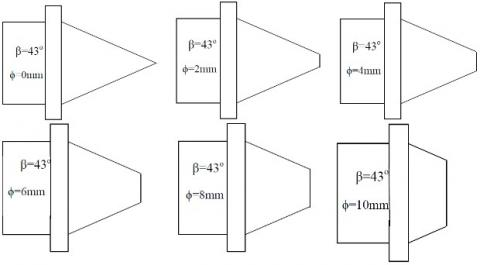Figure 3. A Schematic form of the hot control valve used in tests

In the all of tested vortex tubes β=43o, meanwhile to understand the effect of cone length of throttle valve on the temperature reduction, six different values for ϕ have been taken, i.e. ϕ= 0, 2, 4, 6, 8 and 10mm are experimented by using a constant working tube with length of L = 250 mm.

3. Numerical Simulation

3.1 Governing equations

The compressible turbulent and highly rotating flow inside the vortex tube is assumed to be three-dimensional, steady state and employs the standard k-ε turbulence model on basis of finite volume method. Rafiee and Rahimi  showed the k-ε model can be selected to simulate the effect of turbulence inside the computational domain of vortex tube. Consequently, the governing equations are arranged by the conservation of mass, momentum and energy equations, which are given by:

The equation for conservation of mass, or continuity equation, can be indicated as follows:

$\frac{\partial \rho}{\partial t}+\nabla \cdot(\rho \vec{v})=S_{m}$   (2)

The flow field in this investigation has been assumed ‘steady state’ and term Sm is the mass added to continuous domain from other domains.

Momentum equation:

$\frac{\partial}{\partial x_{j}}\left(\rho u_{i} u_{j}\right)=-\frac{\partial p}{\partial x_{i}}$$+\frac{\partial}{\partial x_{j}}\left[\mu\left(\frac{\partial u_{i}}{\partial x_{j}}+\frac{\partial u_{j}}{\partial x_{i}}-\frac{2}{3} \delta_{i j} \frac{\partial u_{k}}{\partial x_{k}}\right)\right]$$+\frac{\partial}{\partial x_{j}}\left(-\bar{\rho} \bar{u}_{i}^{\prime} \bar{u}_{j}^{\prime}\right)$   (3)

Energy equation:

$\frac{\partial}{\partial x_{i}}\left[u_{i} \rho\left(h+\frac{1}{2} u_{j} u_{j}\right)\right]=$$\frac{\partial}{\partial x_{j}}\left[k_{e f f} \frac{\partial T}{\partial x_{j}}+u_{i}\left(\tau_{i j}\right)_{e f f}\right] (4) Since we determined the working fluid is an ideal gas, then the compressibility effect must be considered as below: p=\rho R T (5) The turbulence kinetic energy (k) and the rate of dissipation (ε) are obtained from the following equations: \frac{\partial}{\partial t}(\rho k)+\frac{\partial}{\partial x_{i}}\left(\rho k u_{i}\right)=\frac{\partial}{\partial x_{j}}\left[\left(\mu+\frac{\mu_{t}}{\sigma_{k}}\right) \frac{\partial k}{\partial x_{j}}\right]$$+G_{k}+G_{b}-\rho \varepsilon-Y_{M}$   (6)

$\frac{\partial}{\partial t}(\rho \varepsilon)+\frac{\partial}{\partial x_{i}}\left(\rho \varepsilon u_{i}\right)=\frac{\partial}{\partial x_{j}}\left[\left(\mu+\frac{\mu_{t}}{\sigma_{\varepsilon}}\right) \frac{\partial \varepsilon}{\partial x_{j}}\right]$$+C_{1 \varepsilon} \frac{\varepsilon}{k}\left(G_{k}+C_{3 \varepsilon} G_{b}\right)-C_{2 \varepsilon} \rho \frac{\varepsilon^{2}}{k}$  (7)

In these equations, Gk, Gb, and YM represent the generation of turbulence kinetic energy due to the mean velocity gradients, the generation of turbulence kinetic energy due to buoyancy and the contribution of the fluctuating dilatation in compressible turbulence to the overall dissipation rate, respectively. C and C  are constants. σk and σε are the turbulent Prandtl numbers for k and ε also. The turbulent (or eddy) viscosity, µt , is computed as follows:

$\mu_{t}=\rho C_{\mu} \frac{k^{2}}{\varepsilon}$   (8)

where, Cμ is a constant. The model constants C, C, Cμ, σk and σε have the following default values: C = 1.44, C = 1.92, Cμ = 0.09, σk = 1.0, σε = 1.3.

3.2 3D CFD model

The 3D CFD mesh grid is shown in Fig. 4. In this model a regular organized mesh grid has been used. All radial line of this model of meshing has been connected to the centerline and the circuit lines have been designed organized from wall to centerline. So, the volume units that have been created in this model are regular cubic volumes. This meshing system helps the computations to be operated faster than the irregular meshing, and the procedure of computations have been done more precisely.

## 4.jpg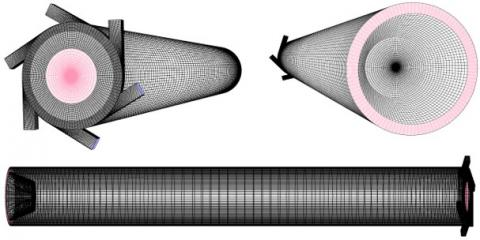Figure 4. 3D CFD model of vortex tube

3.3 Boundary conditions

Boundary conditions for this study have been indicated in Figure 5. The inlet is modeled as pressure inlet. The inlet temperature and the pressure inlet are fixed to 294.2 K and 2.5 bar respectively according to experimental conditions. A no-slip boundary condition is used on all walls of the system. The cold and hot exits boundary condition can be considered as pressure-far-field.

## 5.jpg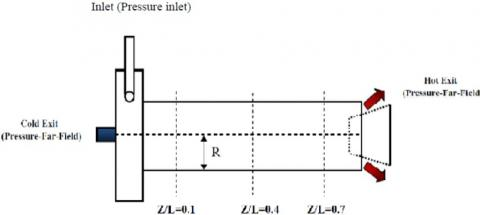Figure 5. A schematic form of boundary conditions

A vortex tube usually works in the ambient condition and for change in the cold mass fraction one need to change the area of hot exit. The computations in this study utilize a pressure correction based iterative SIMPLE algorithm for discretising the convective transport terms. A compressible form of the Navier-Stokes equation along with the standard k-ε model by second order upwind for momentum, turbulence and energy equations has been used to simulate the phenomenon of flow pattern and temperature separation in a vortex tube with  straight inlet nozzles operating under condition of using different geometries of control valve by using the FLUENTTM software package. The default values of under-relaxation factor are shown in Table 2.

Table 2. Under-relaxation factors used for computations

 Under-Relaxation Factor Value Pressure 0.3 Density 1 Body Force 1 Momentum 0.7 Turbulent Kinetic Energy 0.8 Turbulent Dissipation Rate 0.8 Turbulent Viscosity 1 Energy 1

3.4 Grid independence study

The 3D CFD analysis has been performed for different average unit cell volumes in vortex tube as a computational domain. This is for the reason that removing probable errors arising due to grid coarseness. Therefore, first the grid independence study has been done for a=0.24. As seen in the Fig. 8, at this cold mass fraction the vortex tube achieves a minimum outlet cold gas temperature. Consequently, in the most of the evaluations we use a=0.24 as a special value of cold mass fraction.

The variation of cold exit temperature difference and maximum tangential velocity as the main parameters are shown in Figs. 6 and 7 respectively for different unit cell volumes. Not much major advantage can be seen in reducing of the unit cell volume size below 0.026 mm3; which corresponds to 1148000 cells.

## 6.png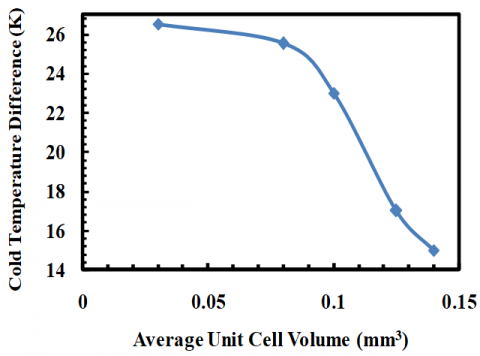Figure 6. Grid size independence study on cold temperature difference at different average unit cell volume

## 7.png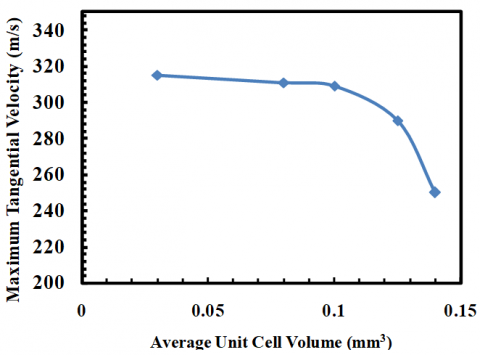Figure 7. Grid size independence study on maximum swirl velocity at different average unit cell volume

4. Results and Discussion

The cold mass fraction and the cold temperature difference between inlet flow and cold stream are two effective parameters to expose the operational characteristics for the vortex tube refrigerator. Cold temperature difference (or temperature reduction) shows the cryogenic performance and can be defined as below:

$\Delta {{T}_{c}}={{T}_{i}}-{{T}_{c}}$  (9)

The experimental results obtained from the tests, which involve the effect of cone length of throttle valve, number of nozzle intakes and the pressure at the inlets on the vortex tube performance, are presented in this section.

4.1 Cone length of throttle valve

## 8.png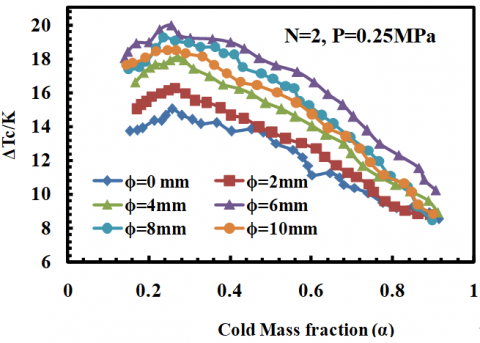Figure 8. Influence of the length of throttle valve on the cold temperature difference

Typical values mentioned above have been employed to investigate the performance of the vortex tube refrigerator with different cone lengths of throttle valve. So far experimental investigation towards the cone length of throttle valve and its effect on vortex tube performance has not been done but the importance of this object can be regarded as an interesting theme of research so that the machine would operate in the way that maximum cooling effect or maximum refrigeration capacity is provided. To expose the effect of cone length of throttle valve on the temperature reduction, six different cone lengths of throttle valve i.e. ϕ=0, 2, 4, 6, 8 and 10 mm are tested by using a constant working tube with length of L=250 mm. The number of nozzle intake is N=2. Fig. 8 shows the variation in ΔTc for cone length of throttle valve at specific cold mass fraction with inlet pressure of 0.25 MPa.

As seen in Fig. 8, the development trend of ΔTc for six different cone lengths of throttle is almost similar. The ΔTc increases with the increase of cold mass fraction up to about α=0.24 and achieves there a maximum value. Thereafter cold temperature difference decreases with the further increase of the cold mass fraction. In Figure 8, it can be seen that, the cone length of throttle valve should be neither too large nor too small and ϕ=6 mm is the best candidate for achieving the highest ΔTc. The highest ΔTc is 20.21 K for ϕ=6 mm at a cold mass fraction of about 0.24, higher than that of ϕ=0 mm around 33.18 % at the near cold flow fraction. It presents that the vortex tube performance can be ameliorated by using truncated cone, and there is an optimum value of cone lengths for obtaining the highest cryogenic performance.

There is a clear decrease of ΔTc for ϕ=8 mm compared with the values for ϕ=6 mm and the difference of ΔTc between them is about 0.8 K at the same cold mass fraction. It shows that the cone lengths of control valve should be a small value not more than 6 mm in our experiment.

4.2 Number of nozzle intakes in vortex tube with truncated cone

On basis of the conclusions for straight nozzles, some reported that increasing the number of nozzles can produce a strong swirling flow field inside the vortex tube and consequently help to improve the temperature separation procedure whereas, some researchers (Yilmaz et al. ) said that the vortex tubes performance decreases with the increase of the nozzles number due to the development of the turbulent flow filed. To indicate the effect of the intake number on the cryogenic capacity of vortex tubes with truncated cone, N=2, 3, 4 and 6 nozzles are investigated at inlet pressure of 0.25 MPa with constant parameters ϕ=6 mm and L=250 mm. Figure 9 indicates the effect of N = 2, 3, 4 and 6 nozzles on the ΔTc of the vortex tube refrigerator with straight nozzles.

## 9.png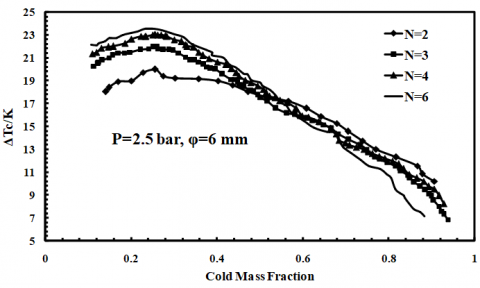Figure 9. Effect of the number of nozzle on the cold temperature difference

As seen in Figure 9, the variation of cold temperature difference corresponding to N=6 has a severe gradient as function of cold mass fraction compared with the mild change for N=2. Consequently, it can be said that increasing the number of nozzles will increase the sensitivity of ΔTc. Also this Figure indicates that the cryogenic ability will increase with the increase of intake number.

4.3 Inlet pressure

## 10.png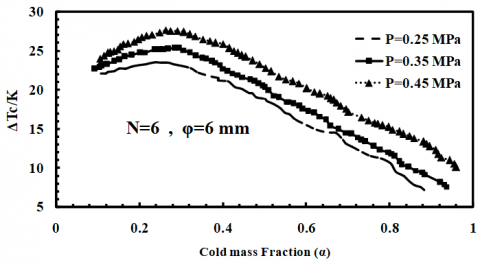Figure 10. Cold temperature difference as functions of the cold mass fraction with various pressures

Figure 10 shows the effects of inlet pressure on the cold temperature difference at the inlet pressures of 2.5, 3.5, 4.5 bar. It is clear that the cold temperature difference is improved by increasing in the inlet pressure. Also the maximum cold temperature difference takes place in the vicinity of α = 0.24 for all inlet pressures of 2.5, 3.5 and 4.5 bar.

Stephan et al.  carried out an approximate investigation for geometrically similar vortex tubes and reported that the ratio of the actual temperature drop of the cold gas that exits from exhaust to the maximum temperature difference ΔTc/(ΔTc)max can be defined as function of  cold mass fraction as below:

$\frac{\Delta {{T}_{c}}}{\Delta {{T}_{c,\max }}}=f(\alpha )$  (10)

In this equation ΔTc is the temperature difference, (ΔTc)max is the maximum temperature drop and α is the cold mass fraction and is varied in range from 0 to 1. To investigate the similarity relation for the vortex tube with truncated cone control valve, tests are conducted for a typical vortex tube. The geometrical characteristics of vortex tubes are indicated in Table 3. The similarity relation ΔTc/(ΔTc)max as a function of α can be taken and indicated in Figure 11. It can be introduced as below:

\begin{align} & \frac{\Delta {{T}_{c}}}{\Delta {{T}_{c,\max }}}=41.27{{\alpha }^{6}}-130.7{{\alpha }^{5}}+157.9{{\alpha }^{4}} \\ & -88.23{{\alpha }^{3}}+20.18{{\alpha }^{2}}-1.063\alpha +0.877 \\\end{align}  (11)

As seen in Figure 11, the ratio of ΔTc/(ΔTc)max for vortex tubes with truncated cone is independent of the inlet pressures, and can be reported as a function of the cold mass fraction.

To confirm the similar relation for our vortex tube with truncated cone, results by Hilsch  and Stephan et al.  are applied for comparison and the results of this comparison is presented in Figure 12. The geometrical parameters of the vortex tube in their experimental investigations are shown in Table 3.

Table 3. Geometrical characteristics of vortex tubes used by some researchers

 Length of working tube Diameter of working tube Diameter of cold exit Number of nozzle Control valve Present Work 250 mm 18mm 9mm 6 Truncated cone Hilsch  300mm 9.2mm 2.6mm 1 N/A Stephan et al  352mm 17.6mm 6.5mm 1 Cone

4.4 Comparisons between CFD results and experimental data

Figure 13 shows the results from the CFD simulation compared to the experimental data. Figure 13 show that the 3D CFD has good agreement with the experimental results. All comparisons between the CFD model and the experimental data are reported in terms of the cold mass fraction. The comparison shows that the 3D CFD results can well predict the experimental results. The difference between experimental data and simulated results at cold mass fraction 0.24 are presented in Table 4.

Table 4 proves that, in this investigation, CFD results concur well with experimental data. Deviation of the predicted and measured values for the cold temperature difference is less than or equal to 4.2%. Finally, the contour of temperature distribution inside the vortex tube is shown as Figure 14.

## 11.png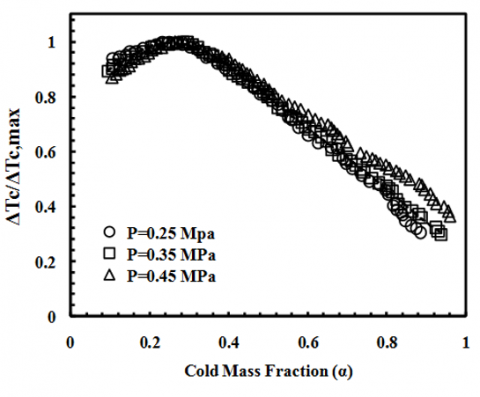Figure 11. Non-dimensional cold temperature difference versus cold mass fraction

## 12.png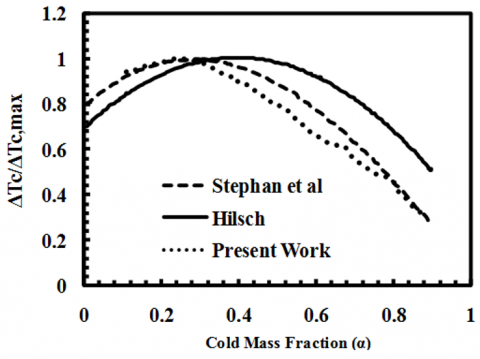Figure 12. Comparison of similarity relations obtained by different works

## 13.jpgFigure 13. Numerical validation with experimental results for different model of ϕ

## 14.jpg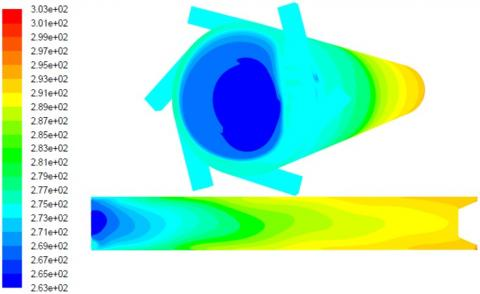Figure 14. Contours of total temperature of the optimum model

Table 4. The difference between experimental data and simulated results at cold mass fraction 0.24  and P=0.45 MPa

 Model ΔTc Experimental (K) ΔTc CFD (K) Difference between experimental and CFD results (%) ϕ=0mm 18.25 18.12 0.7% ϕ=2mm 21.55 20.88 3.2% ϕ=4mm 23.15 22.2 4.2% ϕ=6mm 27.21 26.8 1.5% ϕ=8mm 25.5 24.6 3.5% ϕ=10mm 26.15 25.2 3.6%
5. Conclusions

The influence of the truncated cone length, the inlet pressure at nozzle intakes and the number of nozzle intake on the cryogenic performance was studied experimentally for a vortex tube and the major conclusions can be summarized as below:

(1) The vortex tube performance can be improved by employing truncated cone control valve and the tests illustrated that we have an optimum model between ϕ =0 mm and ϕ=10mm. The tests show that the cone length of throttle valve should be small and not more than 6 mm under our experimental tests so there is an optimum cone length of throttle valve for achieving the highest possible refrigeration performance. The experimental results indicate that the 6mm cone length of throttle valve yields the highest cold temperature reduction, which exceeds the cone one by about 33.18 %.

(2) The influence of nozzle inlets on the cryogenic performance relies on the cold mass fraction. Increasing the number of nozzles leads to a severe sensitivity of ΔTc with the variation of the cold mass fraction and achieves the highest possible cold temperature drop ΔTc at lower cold mass fraction as well.

(3) The ratio of actual cold temperature difference to the maximum temperature reduction, ΔTc/(ΔTc)max, for the vortex tube with truncated cone control valve can be presented as a function of the cold mass fraction.

(4) The purpose of this investigation was to create a 3D CFD model of a simple vortex tube for employ as a design tool in optimizing vortex tube parameters and geometries. The model was developed using a three-dimensional (3D) steady model that utilized the standard k-epsilon turbulence equations. The comparison between the 3D CFD results and the measured experimental data yielded promising results relative to the model’s capability to predict the energy separation. Deviation of the predicted and measured values for the cold temperature difference is less than or equal to 4.2% (at cold mass fraction of 0.24).

Nomenclature

D      Diameter of vortex tube [mm]

k       Turbulence kinetic energy [m2 s-2]

L       Length of vortex tube [mm]

r       Radial distance from the centerline [mm]

T       Temperature [K]

Ti      Inlet gas temperature [K]

Z       Axial length from nozzle cross section [mm]

L       Length (m)

dc    Diameter of cold orifice [mm]

$\dot{m}$      mass flow rate [kg s-1]

R*     The radius of vortex-chamber [mm]

S       The width of a nozzle [mm]

Greek Symbols

T    Temperature difference [K]

α       Cold mass fraction

ϕ       Cone length [mm]

β       Cone angle

Subscripts

in     Inlet

c       Cold

h       Hot

t        Total

References

 Ranque, G.J. (1933). Experiments on expansion in a vortex with simultaneous exhaust of hot air and cold air. Le J. de Physique et le Radium, 4: 112-114.

 Hilsch, R. (1947). The use of expansion of gases in a centrifugal field as a cooling process. Rev. Sci. Instrum, 18: 108-113. http://dx.doi.org/10.1063/1.1740893

 Aydin, O., Baki, M. (2006). An experimental study on the design parameters of a counter flow vortex tube. Energy, 31: 2763–2772. https://doi.org/10.1016/j.energy.2005.11.017

 Kurosaka, M. (1982). Acoustic streaming in swirling flow and the Ranque-Hilsch (vortex-tube) effect. Journal of Fluid Mechanics, 124: 139-172. https://doi.org/10.1017/S0022112082002444

 Stephan, K., Lin, S., Durst, M., Seher, F., Huang, D. (1983). An investigation of energy separation in a vortex tube. International Journal of Heat and Mass Transfer, 26(3): 341-348. https://doi.org/10.1016/0017-9310(83)90038-8

 Dincer, K., Baskaya, S., Uysal, B.Z. (2008). Experimental investigation of the effects of length to diameter ratio and nozzle number on the performance of counter flow Ranque–Hilsch vortex tubes. Heat Mass Transfer, 44: 367–373. https://doi.org/10.1007/s00231-007-0241-z

 Ahlborn, B.K., Gordon, J.M. (2000). The vortex tube as a classic thermodynamic refrigeration cycle. Journal of Applied Physics, 88: 3645-3653. https://doi.org/10.1063/1.1289524

 Akhesmeh, S., Pourmahmoud, N., Sedgi, H. (2008). Numerical study of the temperature separation in the Ranque-Hilsch vortex tube. American Journal of Engineering and Applied Sciences, 3: 181-187. http://dx.doi.org/10.3844/ajeassp.2008.181.187

 Aljuwayhel, N.F., Nellis, G.F., Klein, S.A. (2005). Parametric and internal study of the vortex tube using a CFD model. Int. J. Refrig, 28: 442-450. https://doi.org/10.1016/j.ijrefrig.2004.04.004

 Skye, H.M., Nellis, G.F., Klein, S.A. (2006). Comparison of CFD analysis to empirical data in a commercial vortex tube. Int. J. Refrig, 29: 71-80. https://doi.org/10.1016/j.ijrefrig.2005.05.004

 Rafiee, S.E., Rahimi, M., Pourmahmoud, N. (2013). Three-dimensional numerical investigation on a commercial vortex tube based on an experimental model - part i: optimization of the working tube radius. Int. J. Heat and Tech, 31(1): 49-56. https://doi.org/10.18280/ijht.310107

 Chang, H.S. (2006). Experimental and Numerical Studies in a Vortex Tube. Journal of Mechanical Science and Technology, 20(3): 418-425. https://doi.org/10.1007/BF02917525

 Im, S.Y., Yu, S.S. (2012). Effects of geometric parameters on the separated air flow temperature of a vortex tube for design optimization. Energy, 37(1): 154-160. https://doi.org/10.1016/j.energy.2011.09.008

 Polat, K., Kirmaci, V. (2011). Determining of gas type in counter flow vortex tube using pairwise fisher score attribute reduction method. Int. J. Refrig, 34: 1372-1386. https://doi.org/10.1016/j.ijrefrig.2011.05.010

 Rafiee, S.E., Rahimi, M. (2013). Experimental study and three-dimensional (3D) computational fluid dynamics (CFD) analysis on the effect of the convergence ratio, pressure inlet and number of nozzle intake on vortex tube performance–Validation and CFD optimization. Energy, 63: 195-204. https://doi.org/10.1016/j.energy.2013.09.060

 Yilmaz, M., Kaya, M.E.H.M.E.T., Karagoz, S., Erdogan, S. (2009). A review on design criteria for vortex tubes. Heat and mass transfer, 45(5): 613-632. https://doi.org/10.1007/s00231-008-0447-8